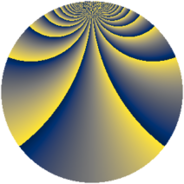# Properties

 Label 2240.2.beLevel $2240$ Weight $2$ Character orbit 2240.be Rep. character $\chi_{2240}(559,\cdot)$ Character field $\Q(\zeta_{4})$ Dimension $184$ Sturm bound $768$

# Related objects

## Defining parameters

 Level: $$N$$ $$=$$ $$2240 = 2^{6} \cdot 5 \cdot 7$$ Weight: $$k$$ $$=$$ $$2$$ Character orbit: $$[\chi]$$ $$=$$ 2240.be (of order $$4$$ and degree $$2$$) Character conductor: $$\operatorname{cond}(\chi)$$ $$=$$ $$560$$ Character field: $$\Q(i)$$ Sturm bound: $$768$$

## Dimensions

The following table gives the dimensions of various subspaces of $$M_{2}(2240, [\chi])$$.

Total New Old
Modular forms 800 200 600
Cusp forms 736 184 552
Eisenstein series 64 16 48

## Trace form

 $$184q + O(q^{10})$$ $$184q + 8q^{11} - 16q^{21} - 8q^{29} + 24q^{35} + 16q^{39} - 8q^{49} + 32q^{51} - 8q^{65} - 48q^{71} - 136q^{81} - 24q^{85} + 48q^{91} - 40q^{99} + O(q^{100})$$

## Decomposition of $$S_{2}^{\mathrm{new}}(2240, [\chi])$$ into newform subspaces

The newforms in this space have not yet been added to the LMFDB.

## Decomposition of $$S_{2}^{\mathrm{old}}(2240, [\chi])$$ into lower level spaces

$$S_{2}^{\mathrm{old}}(2240, [\chi]) \cong$$ $$S_{2}^{\mathrm{new}}(560, [\chi])$$$$^{\oplus 3}$$